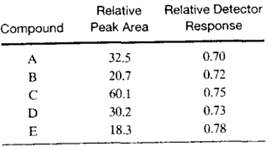### Create an Account

Already have account?

### Forgot Your Password ?

Home / Questions / Determine the concentration of species in a sample using the peak areas and relative detec...

# Determine the concentration of species in a sample using the peak areas and relative detector responses for the five gas chromatographic peaks given in the following table Use the area normalization

Determine the concentration of species in a sample using the peak areas and relative detector responses for the five gas chromatographic peaks given in the following table. Use the area-normalization method described in Problem 27-25.

Also shown are the relative responses of the detector. Calculate the percentage of each component in the mixture.Problem 27-25

One method for quantitative determination of the concentration of constituents in a sample analyzed by GC is the area-normalization method. Here, complete elution of all of the sample constituents is necessary. The area of each peak is then measured and corrected for differences in detector response to the different eluates. This correction involves dividing the area by an empirically determined correction factor. The concentration of the analyte is found from the ratio of its corrected area to the total corrected area of all peaks. For a chromatogram containing three peaks, the relative areas were found to be 16.4, 45.2, and 30.2 in the order of increasing retention time. Calculate the percentage of each compound if the relative detector responses were 0.60, 0.78.and 0.88. respectively.

Jun 23 2020 View more View Less

#### Answer (Solved)Subscribe To Get Solution